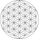750 lượt xem
750
Smoothed BOP to try and find dark pool activity. Only works in charts with working volume!

Credits go to LazyBear for some coding on the plotting and Igor Livshin for the formula.
```// author : SatNam
// credits to LazyBear and Igor Livshin

study(title="SN-BOP")
length=input(14)
lengthSMA=input(50)
PlotEMA=input(true, "Plot SMA?", type=bool)
THL = (high-low) ? .00001 : (high-low)

BuRBoO = (high - open)/(THL)
BeRBoO = (open - low)/(THL)

BuRBoC =(close - low)/(THL)
BeRBoC =(high - close)/(THL)

BuRBoOC = close > 0 ? (close-open)/(THL) : 0
BeRBoOC = close > 0 ? 0 : (open -close)/(THL)

BOP = ((BuRBoO+BuRBoC+BuRBoOC)/3) - ((BeRBoO+BeRBoC+BeRBoOC)/3)
vwBOP = vwma(BOP, length)
barcolor = vwBOP >= 40000 ? green : vwBOP <= -40000 ? red: gray
hline(0)
plot(vwBOP, style=columns, color = barcolor)
plot(PlotEMA?ema(BOP, lengthSMA):na, color=navy, linewidth=2)```AMBRISH
No problem. I'm making an updated version that can be used on any chart and only needs volume information from another chart. This way you could, for instance, get hourly volume information on a FXCM chart using a different chart for volume information.
Phản hồi